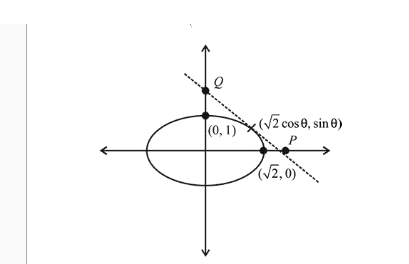# If tangents are drawn to the ellipseQuestion:

If tangents are drawn to the ellipse $x^{2}+2 y^{2}=2$ at all points on the ellipse other than its four vertices then the mid points of the tangents intercepted between the coordinate axes lie on the curve :

1. (1) $\frac{1}{4 x^{2}}+\frac{1}{2 y^{2}}=1$

2. (2) $\frac{x^{2}}{4}+\frac{y^{2}}{2}=1$

3. (3) $\frac{1}{2 x^{2}}+\frac{1}{4 y^{2}}=1$

4. (4) $\frac{x^{2}}{2}+\frac{y^{2}}{4}=1$

Correct Option: , 3

Solution:

Given the equation of ellipse,

$\frac{x^{2}}{(\sqrt{2})^{2}}+y^{2}=1$$\frac{\sqrt{2} \cos \theta x}{2}+y \sin \theta=1$

$P\left(\frac{\sqrt{2}}{\cos \theta}, 0\right)$ and $Q\left(0, \frac{1}{\sin \theta}\right)$

Let mid point be $(h, k)$

$\Rightarrow \quad h=\frac{1}{\sqrt{2} \cos \theta}, k=\frac{1}{2 \sin \theta}$

As $\cos ^{2} \theta+\sin ^{2} \theta=1$

$\therefore \quad \frac{1}{2 h^{2}}+\frac{1}{4 k^{2}}=1$]

Locus is $\frac{1}{2 x^{2}}+\frac{1}{4 y^{2}}=1$